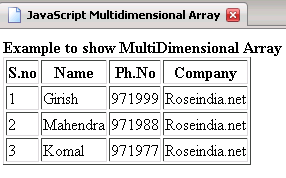Tutorials

# JavaScript array multidimensional

In this Tutorial we want to describe you a code that help you in understanding JavaScript array multidimensional.

In this Tutorial we want to describe you a code that help you in understanding JavaScript array multidimensional.

# JavaScript array multidimensional

In this Tutorial we want to describe you a code that help you in understanding JavaScript array multidimensional. For this we are using JavaScript language, This code illustrate a multidimensional array application used in creating a table. The document. write create a table with 4 columns and 4 rows with a different field name of the  column.

In this code we consider a function multidimensional array that accept table as argument .Inside this function we print a table showing you 'Example to show Multidimensional Array' and print the name of respective columns. The for loop execute the script till the variable x is less than equal to 3.The document. write print the number of columns. In the same way the for loop execute the script and print the number of  rows till variable y is less than equal to 2.The var array instantiate an array object and store the value passed as parameter to it. The var new array instantiate the array object by accepting the arrays object as parameter. Finally a new multidimensional table is created and show the list of different value in the respective column.

JavascriptMultidimensionalArray.html

 ```      JavaScript Multidimensional Array      function multidimensionalArray(table) {   document.write("Example to show    MultiDimensional Array"+"
");   document.write("");    document.write("
S.noNamePh.NoCompany
",x,"",table[x][y],"
\n\  ");   for(var x=1; x<=3; x++) {   document.write("");   for(var y=0; y<=2; y++){   document.write("");   }   document.write("");   }   document.write("");   }   var array1 = new Array("Girish","971999","Roseindia.net");   var  array2 = new Array("Mahendra","971988","Roseindia.net");   var  array3 = new Array("Komal","971977","Roseindia.net");   var newarray = new Array("",array1,array2,array3);   multidimensionalArray(newarray);            ```

Output of the program